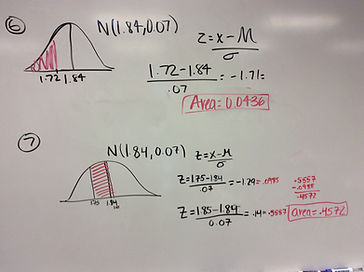## Chapter 5 - Day 11

##### Activity: Normal Distributions Practice

Normal distribution calculations are so vital to our future success in this class, so we spent an extra day doing some practice problems.  Students worked in pairs, and then we had students put solutions on the board.  As a class, we critiqued each solution on the board, being sure to look for (1) correctly labeled and shaded picture, (2) z-score formula and numbers, and (3) correct z-score and area.Then we showed the students how to use the Applet to do the same normal distribution calculations.  No z-score calculations.  Just simply type in the mean and standard deviation, and tell the applet which area you are looking for.  Students were mad.